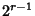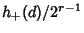## Fundamental Theorem of Genera

Considerproper equivalence classes of forms with discriminantequal to the field discriminant, then they can be subdivided equally intogenera offorms which form a subgroup of the proper equivalence class group under composition (Cohn 1980, p. 224), whereis the number of distinct prime divisors of. This theorem was proved by Gaußin 1801.

References

Arno, S.; Robinson, M. L.; and Wheeler, F. S. ``Imaginary Quadratic Fields with Small Odd Class Number.'' http://www.math.uiuc.edu/Algebraic-Number-Theory/0009/.

Cohn, H. Advanced Number Theory. New York: Dover, 1980.

Gauss, C. F. Disquisitiones Arithmeticae. New Haven, CT: Yale University Press, 1966.